# Improving the Response Time of a Soft Robotic Gripper Using a Heat Sink with Shape Memory Alloy Actuators

Improving the Response Time of a Soft Robotic Gripper Using a Heat Sink with Shape Memory Alloy Actuators
Mechanical and Industrial Engineering Technology, University of Johannesburg, Johannesburg 2028, South Africa

Corresponding Author Email:
kgupta@uj.ac.za
Page:
565-574
|
DOI:
https://doi.org/10.18280/jesa.560406
22 May 2023
|
Revised:
22 June 2023
|
Accepted:
17 July 2023
|
Available online:
31 August 2023
| Citation

OPEN ACCESS

Abstract:

This study focuses on the time response analysis of a nickel titanium (NiTi) shape memory alloy-based soft robotic gripper with variable stiffness, with a particular emphasis on reducing the cooling time, which will ultimately lead to a faster response time. The manufacturing of the shape memory alloy is not covered in this work. The impact of a heat sink comprising a silicone casing and an ethylene glycol thermal compound on the response time was examined using Finite Element Analysis. The cylindrical cross-section of the gripper's finger was created as a three-dimensional model. Numerical analysis was done using Ansys Workbench, and experimental results validated the numerical results with a 1.12% percentage difference. A 36% overall improvement in response time was observed, indicating that the proposed heat sink is capable of acceleration the rate of heat dissipation from the shape memory alloy, and in turn improves the response time of the gripper.

Keywords:

actuator, heat sink, robotic gripper, shape memory alloy, stiffness

1. Introduction

Recently, the global robotics community and researchers have taken a particular interest in the subject of soft robotic grippers based on Shape Memory Alloys (SMAs). They are appropriate for application in a variety of industries due to several advantages that they possess. These include low energy consumption, dexterity, compliance, and compact and lightweight structure . On the other hand, traditional robotic grippers, which often have complex structural elements and control mechanisms, are cumbersome and less compliant in unstructured environments [2-5]. Compared to their conventional rigid counterparts, soft robots are more adaptable and flexible, but these qualities come at the sacrifice of platform stability. Utilizing mechanisms with changing stiffness, this restriction is overcome. Other researchers investigated different types of soft robotic grippers with variable stiffness, such as granular jamming, in which a significant number of tiny granules enclosed within a membrane are pressed together when the membrane collapses under vacuum pressure created within the membrane. Granular jamming-based systems' architecture, however, is complicated because it depends on supplementary vacuum pumps . It was also observed by researchers previously that soft pneumatic actuators, although capable of variable stiffness, their unpredictable performance and their dependency upon compressors for the supply of compressed air or vacuum, limited their practical utility [2, 3]. Other mechanisms with variable stiffness capabilities are the dielectric elastomer actuators. However, they are limited by the amount of actuation force they produce , also they are not easy to fabricate, they require high voltage to operate and their compliance is unreliable .

SMAs belongs to the group of smart materials that possess the capacity to return to their original shape when heated after being distorted [6-8]. This is because they exhibit both shape memory effect (SME) and super elasticity which allows them to regain their original shape after having been distorted [9, 10]. The first discovery of SME was made in 1951 by Chang and Read after experimenting with gold-cadmium (AuCd). This was followed by the discovery of nickel titanium (NiTi) or nitinol SMAs in 1962, and ever since then there has been an extensive commercial use of these materials in different fields including medicine [11, 12]. SME is an effect of phase transformation, giving rise to austenite phase at high temperatures and martensite phase at low temperatures. This allows SMAs to be used as actuators and energy storage devices in soft robotic grippers with variable stiff-ness [13, 14]. The selection of a suitable manufacturing process of SMAs is not an easy task. This is evidenced by a study performed by the study , which involved the machining of a high-temperature Copper (Cu)-Zirconium (Zr) added NiTi SMA with high cyclic stability and transformation temperature. According to the study  these types of SMAs can perform better than ternary and binary SMAs regarding high temperatures and functional fatigue.

With an extensive review of the literature. It has been noted that while some other types of soft robotic grippers may have a more rapid response time, this is achieved at the compromise of the simplistic, compact, lightweight, and cost-effective design of these grippers. As a result, the system becomes more complex, negating many of its benefits related to its structural design. This study proposes a simple design of SMA based soft robotic gripper with variable stiffness. What makes it unique is its ability to improve response time while maintaining the simplistic, compact, lightweight, and cost-effective structural design. It was also discovered that using existing FEM tools such as ANSYS workbench and Autodesk, it is not easy to perform thermal simulation of shape memory materials since most of them do not exist in the materials library of such platforms. Not much simulation of shape memory materials (particularly NiTi SMAs) has been covered in the literature, especially regarding the analysis of the time response.

The present work attempts to demonstrate that while it might sometimes be difficult to procure these materials, the numerical simulation tools may still be used to gain understanding of these materials. Despite substantial research into SMA-based soft grippers with variable stiffness, their poor response time continues to be their major limitation. The primary objective of this study is to investigate methods of improving the response time of shape memory alloy-actuated soft robotic grippers through simulations and experiments, without compromising their simplistic and lightweight design.

2. Methodology

The goal of this study is to examine the time response of a soft robotic gripper based on SMA with varying stiffness. Finite element analysis and experiments were conducted to analyze the response time of a soft robotic gripper actuated by shape memory alloy wires, with and without a proposed heat sink. The system's mathematical model is developed first, followed by a performance analysis of the system. A transient thermal analysis was performed using FEA. In the ANSYS workbench, a 3D model of the gripper's finger's circular cross-section was then analyzed. The first model created and analyzed is of a NiTi SMA without a heat sink.

An integrated SMA wire within the soft robotic gripper's cylindrical finger construction actuates the finger when thermally activated. Because of the force generated by the SMA actuator, the finger then grasps an object. When the Ms temperature reaches 52℃, the finger begins to release an object as the SMA undergoes martensitic reverse phase transformation, and the process is complete when the Mf temperature reaches 42℃. We should be able to monitor the rate of heat transfer through the SMA from the analysis findings, and we should be able to compute the time required to transition from the Ms temperature of the NiTi SMA of 52℃ to its Mf temperature of 42℃. In the absence of any heat sink mechanism, this will be the time required for the embedded NiTi SMA to undergo a complete reverse martensitic phase transformation. This corresponds to the response time, which is the time it takes for the soft robotic gripper's fingers to entirely release an object from their grasp. The heating time is not considered in this work since the time required to heat the SMA to the point of phase change is irrelevant in this context. The time it takes for heat to disperse from the SMA represents a response time limitation. Figure 1 shows a three-dimensional model of a soft robotic gripper developed in Autodesk inventor.

The SMA is generally incorporated within an SMP in SMA-based soft robotic grippers with variable stiffness. The strategy used in this study will be to incorporate an SMA actuator within a compliant shell that will operate as part of the heat sink, removing the requirement for other approaches that would compromise the system's compact and lightweight structural architecture. The numerical results will be validated using experimental data. A NiTi SMA will be heated until the Ms temperature reaches 52℃. The heating method is insignificant in this study because the cooling period is the primary subject of this work. The SMA is heated to the proper temperature using a flame. The response time is affected also by the SMA's size. However, the effect of dimensions on response time was ignored in this work, because if the proposed heat sink proves efficient, it can then be employed with an SMA of any given dimensions. As a result, the experiment was carried out with a 15 mm diameter SMA. SME is observed for a NiTi SMA of lower size at the Ms temperatures described in this work. On the other hand, SME was not expected for the NiTi SMA employed in this study. This is due to its dimensions. The SMA is then allowed to cool down in ambient conditions using free convective heat transfer until a Mf temperature of 42℃ is obtained.

Through a series of trials, the time taken to cool the NiTi SMA from Ms to Mf  temperature is measured; for the sake of this study, this corresponds to the response time of the gripper's fingers upon releasing an object. The NiTi SMA is next coupled with the proposed heat sink, and its cooling time by free convection under ambient conditions is measured. To determine any improvements in response time, its values are compared for SMA with and without the heat sink.

## image004.png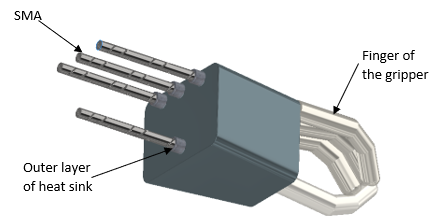Figure 1. 3-D model of the proposed robotic gripper

2.1 Proposed finger actuation mechanism

When thermally agitated, the embedded SMA wires contract. This serves as a driving force for the finger. Electrical current can be used to provide thermal stimulation. To help with stiffness modulation, the SMA is placed within a compliant material. The SMA wires bend the fingers, commencing the gripping movement, and are guided by a collection of pullies. The pulleys help keep the SMA in place and to guide it as flexes and contracts. The parallel configuration of the SMAs is meant to maximize grabbing force and strain generation. The SMAs should regain their original configuration after cooling. To speed up cooling, a heat sink is utilized, with the SMA placed within the heat sink mechanism embedded within the finger structure. Finger extension is aided by small compression springs within the finger structure. The spring is meant to aid in speeding up the rate of extension of the finger. Figure 2 shows a cross-section of the finger construction and proposed actuation mechanism.

## image005.pngFigure 2. Representation of the finger structure

2.2 Numerical modeling

The soft robotic gripper's fingers have a circular cross-section. As a result, the analytical model used in the FEA is that of heat transfer through composite cylindrical bodies. Because all the fingers have the same dimensions and arrangement, the analysis will be performed on a single finger, and the results will be the same for each finger. Because the temperature distribution is from the embedded SMA outwards across the circular cross-section of the finger structure, the analysis focuses on the circular cross-section of the finger.

Several criteria were considered when determining the geometry of the heat sink. The first thing to notice is the ease of manufacture. Due to a lack of access to complex molding machinery, a simple layout was chosen because molding will be the primary way of creating the outer shell of the finger structure, which also serves as the outer shell of the heat sink. The second factor is that molding intricate configurations with silicone rubber proved problematic. As a result of the absence of geometric complexity, a simpler cylindrical configuration was chosen.

2.3 Modelling

The formulation of the model assumed a one-dimensional radial heat transfer in a cylindrical element, representing heat dissipation from an embedded SMA wire. An initial temperature, corresponding to the Ms temperature of NiTi SMAs of 52℃ was applied. An ambient temperature of 22℃ was applied. Convective cooling, with a film coefficient or coefficient of heat transfer of 13.75 (W/m2 K) was applied. Two considerations had an impact on the suggested heat sink's design and material choices. The ease of fabrication while still maintaining effective heat dissipation, was the first factor to be considered. The availability of the materials to be employed was the second factor. Silicone was found to be a suitable match for the casing. Ethylene glycol was selected as a suitable thermal compound due to its thermal properties.

As demonstrated by Figures 3(a) and (b), heat transfer through composite walls happens across two layers with varying dimensions and material qualities that are in thermal contact.

## image006.png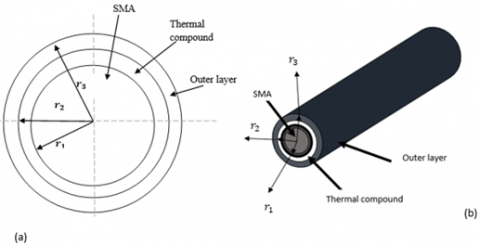Figure 3. Cross-section of a coaxial composite cylindrical element. (a) 2D view, (b) 3D view

2.4 Heat transfer through single layered cylindrical bodies

Given that we are dealing with a cylindrical body, Fourier's law in radial coordinates yields differential Eq. (1).

$q_r=-\frac{K A \partial T}{\partial r}$                           (1)

where, qr is the rate of heat transfer in the radial direction (J/s), K is the thermal conductivity (W/mK), A is the area (m2), and $\partial_r$ is the differential radial thickness of the element (mm). A cylinder has a circular cross-section with the given length L and radius (r). Eq. (2) represents the circumferential region across which heat will flow.

$A=2 \pi r L$                           (2)

where, A is the circumferential area across which heat will flow (m2), r is the radius (m), and L is the length (m). Substituting Eq. (2) into Eq. (1), we obtain Eq. (3).

$q_r=-K 2 \pi r L \frac{\partial T}{\partial x}$                           (3)

When the variables in Eq. (3) are separated, Eq. (4) is obtained.

$\frac{q_r \partial r}{2 \pi r L}=-K \partial T$                           (4)

When integrating, we obtain Eq. (5).

$\frac{q_r}{2 \pi L} \int \frac{d r}{x}=-K \int d T$                           (5)

where, r=ri, r=r0, and T=Ti, T=T0 represent boundary conditions. ri is the inner radius r0 is the outer radius, Ti is the inner temperature.and T0 is the outer temperature.

When the integrals are evaluated, Eq. (6) is obtained.

$\frac{q_r}{2 \pi L}[l n]_{r_i}^{r_0}=-K[T]_{T_i}^{T_0}$                         (6)

where, r=ri, r=r0, T=Ti, and T=T0 represent the boundary conditions. ri being the inner radius of the cylindrical cross-section of the finger structure (m), r0 is the outer radius of the cylindrical cross-section of the finger structure (m), Ti is the inner temperature of the finger (K), and T0 is the outer temperature of the finger (K).

When integrating over the boundary conditions we obtain Eq. (7).

$\frac{q_r}{2 \pi L}\left(\ln r_0-\ln r_i\right)=-K\left(T_0-T_i\right)$                         (7)

The left-hand side of Eq. (7) is simplified to obtain Eqs. (8), (9), and (10), with boundary conditions T0=22℃ and Ti=52℃.

$\frac{q_r}{2 \pi L} \ln \frac{r_0}{r_i}=-K\left(T_0-T_i\right)$                         (8)

$q_r=-\frac{2 \pi L K\left(T_0-T_i\right)}{\ln \frac{r_0}{r_i}}$                         (9)

$q_r=\frac{2 \pi L K\left(T_i-T_0\right)}{\ln \frac{r_o}{r_i}}$                         (10)

2.5 Heat transfer through multilayered cylindrical bodies

Consider Figure 4, which represents the three layers that comprise the finger structure of the gripper. These being the NiTi SMA, thermal compound, and the outer silicone casing. Let r0, r1, and r2 be the radii (m) of the layers with k1 and k2 respectively being the average thermal conductivities (W/mK) of the layers' materials, Tb being the inner temperature (K), and hb being the heat transfer coefficient (W/m2 K). Ta (K) is the outside temperature, and ha (W/m2 K) is the heat transfer coefficient. The interface temperatures are T0, T1, and T2 (K). R0, R1, and R2 are thermal resistivities (J/s). As a result, the heat flow rate through the coaxial cylindrical finger construction with a sequence of resistances may be computed.

## image017.png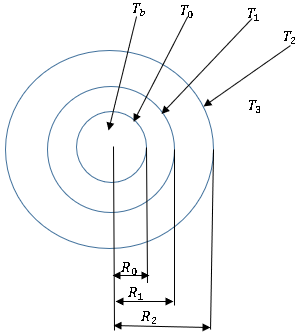Figure 4. Heat flow through a composite cylindrical body

When considering a hollow cylindrical body with very thin dimensions, that has a radial distance r and an elemental differential radial thickness $\partial_r$. If r is sufficiently tiny in relation to r, the inner and outer surfaces of the thin cylindrical body may have the same area. If the body is large enough, the heat flow through the ends may be deemed negligible, removing any temperature dependence on the axial coordinates.

Figure 5 depicts a multi-layered cylindrical body with one directional heat flow from Ti to T0 in a radial manner over a circular cross section, with boundary conditions r=ri, r=r0, T= Ti, and T=T0. R is the thermal resistance or thermal resistivity (K/W) of a material, which is measured in terms of temperature difference . Eq. (11) represents thermal resistance.

## image018.png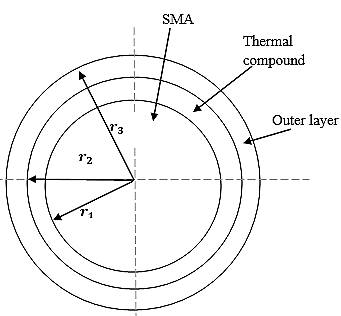Figure 5. Cross section of the finger of the robotic gripper

$R_{T h}=\frac{T_i-T_0}{q_r}$                       (11)

where, $R_{T h}$ represents the thermal resistivity, $q_r$ is the heat flow rate, and Tt, T0 represent temperature boundary conditions.

When Eq. (10) is substituted into Eqs. (11) and (12) are obtained.

$R_{T h}=\frac{\ln \frac{r_o}{r_i}}{2 \pi L K}$                       (12)

When considering Figure 5, which depicts a cylindrical cross section of the robotic gripper's finger. Because heat flows from the interior to the outside surface, across materials with varying thermal and material properties, the materials individual thermal resistivity can be expressed by Eqs. (13) and (14).

$R_{\text {Compound }}=\frac{\ln \frac{r_2}{r_1}}{2 \pi L K_{\text {compound }}}$                       (13)

$R_{\text {OuterLayer }}=\frac{\ln \frac{r_3}{r_2}}{2 \pi L K_{\text {OuterLayer }}}$                       (14)

where, RCompound represents the thermal compound layer thermal resistance (K/W), Kcompound represents the thermal compound layer thermal conductivity (W/mK), r1 is the inner radius (m), r2 is the outer radius (m). ROuterLayer is the outer silicone casing thermal resistance (K/W), KOuterLayer is the outer silicone casing thermal conductivity (W/mK), r2 is the inner radius (m), and r3 is the outer radius (m) of the outer casing.

When considering the flow of heat from the interior surface to the exterior surface, the two resistance expressions can be added together to obtain Eq. (15).

$q_r=\frac{\Delta T}{R_{\text {Compound }}\quad+R_{\text {OuterLayer }}}$                       (15)

where, qr represents the overall heat transfer rate in radial coordinates through a multi-layered cylindrical cross-section of the finger of the robotic gripper (J/s), $\Delta T$ is the total temperature difference (K).

2.7 Finite element analysis procedure

Ansys workbench was used to create a 3D model of the gripper's finger's circular cross-section. Because NiTi SMA does not exist in Ansys workbench's engineering materials library, it was constructed using the user defined material subroutine, using the material properties of nitinol SMAs as given in Table 1 and Table 2 give the properties that give Nitinol its unique characteristics.

Table 1. Physical properties of Nitinol SMA

 Melting Point 1300℃ Density 6.45 cm3 Thermal Conductivity Austenite 18 W/mK Martensite 8.6 W/mK Specific Heat 0.837 J/g℃

Table 2. Mechanical properties of Nitinol SMA 

 Young’s Modulus (GPa) Austinite ~83 Martensite ~28 - 41 Yield Strength (MPa) Austinite 195 - 690 Martensite 70 - 140 Ultimate Tensile Strength (MPa) Fully annealed 895 Work hardened 1900 Poisson’s ratio 0.33 Elongation at Failure (%) Fully annealed 25 - 50 Work hardened 5 - 10

Following the production of Nitinol with the specified qualities, the model was revised, and the necessary materials were allocated. The result was a mixed mesh. The mesh in Figure 6 was improved to include 2864 elements and 3281 nodes, with physical preference set to CFD and solver set to fluent. To establish mesh convergence, deafferent mesh sizes were generated. The mesh size was then decreased until the results remained somewhat consistent. At this point the mesh was refined no further as convergence had been established.

## image027.png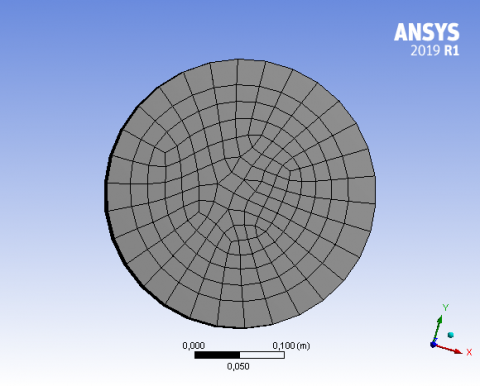Figure 6. Mesh generated in ANSYS for thermal analysis of cylindrical cross section of Nitinol SMA

Table 3. Properties of commonly used shape memory materials

 Parameters Value SMA martensitic elastic modulus (Em) SMA austenitic elastic modulus (EA) 28-41 GPa ~83 GPa SMA martensitic start temperature (Ms) SMA martensitic finish temperature (Mf) 52℃ 42℃ SMA austenitic start temperature (As) SMA austenitic finish temperature (Af) 68℃ 78℃ SMA resistance per meter 55$\Omega$ SMP glassy elastic modulus (EG) SMP rubbery elastic modulus (ER) 0.57 MPa–3.7 MPa 10 MPa SMP glass transition temperature (EG) 55℃ PDMS temperature range PDMS elastic modulus (EPDMS) 200℃ 1.84MPa

Table 4. Typical values of the convective heat transfer coefficient 

 Type of Convection Convective Heat Transfer Coefficient (h) Btu/(h-ft2-R) W/(m2-K) Air, free 1-5 2.5-25 Air, forced 2-100 10-500 Liquids, forced 20-3000 1000-15000 Boiling water 500-5000 2500-25000 Condensing water vapor 1000-20000 5000-100000

Following that, a transient thermal analysis with predetermined boundary conditions was performed. The boundary conditions were set at 52℃ for the beginning temperature and 22℃ for the ambient temperature. The initial temperature corresponds to the Ms temperature of NiTi SMAs as shown in Table 3 . At ambient conditions, a film coefficient or coefficient of heat transfer was set at 13.75 (W/m2 K), and this value was used as the average coefficient of heat transfer in ambient conditions. Table 4 shows typical values of convective heat transfer coefficients.

Convective heat transfer is represented by Eq. (16) as the constant of temperature difference between an item and the surrounding medium.

$Q_{\text {conv }}=h A\left(T_{\infty}-T_s\right)$                                (16)

where, Qconv is the convective heat transfer rate, (h) is the convective heat transfer coefficient(W/m2 K), (A) is the surface area of the object being cooled or heated (m2), T is the surrounding fluid's bulk temperature, and Ts is the surrounding fluid's surface temperature. A 3D model of a NiTi SMA connected with a heat sink consisting of a 0.5 mm thick silicone casing and a thermal compound layer was also created as represented by Figure 7. Tables 5 and 6 show the material data of silicone and thermal compound, respectively.

## image029.png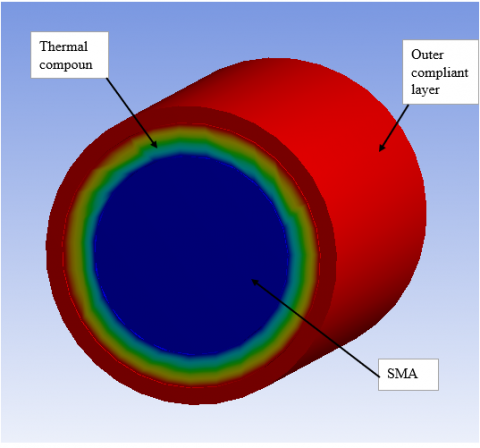Figure 7. 3D model of SMA and heat sink

Table 5. Silicone rubber properties

 Property Value Thermal conductivity 159 W/m K Density 1240 kg/m3 Specific heat 768 J/kg K

Table 6. Properties of ethylene glycol thermal compound

 Property Value Thermal conductivity 159 W/m ℃ Density 1111.4 kg/m3 Specific heat 2415 J/kg ℃

When selecting a material for use in soft robotic grippers with variable stiffness, keep in mind that commonly used materials have viscoelastic properties . Polymers are resistant to deformation due to their elastic properties. This indicates that they can restore to their predetermined configurations after distortion. They also have viscous properties, which means that the original configuration may not always be preserved after distortion. The combination of these two features gives polymers their viscoelastic behavior . Viscoelasticity is critical in soft robotic applications. As a result, materials having the greatest viscoelasticity are the most preferred. The most used viscoelastic materials in soft robotic applications are urethanes and polyacrylates.

In high cycle loading applications, materials with low viscoelasticity, such as silicone, are typically used. They are also advantageous in working settings characterized by high elastic resilience . High elastic resilience and cycle loading are both present in soft robotic grippers with variable stiffness. Also, silicone rubber exhibits excellent thermal stability and is often used in high temperature working conditions .

As a result, silicone rubber is the best material to utilize. Silicone is also favorable for the construction of the soft gripper's fingers because it is easily molded . Some of the material qualities of silicone rubber are shown in Table 5.

Following the creation of the model, appropriate materials were allocated, and a mesh was created. Mesh refinement was carried out using CFD physical preference and the fluent solver, yielding the mesh shown in Figure 8. One of the motivations for selecting a specific mesh or element type in FEA is to reduce computation time while preserving accuracy. The intricacy of the model generated may influence the choice of mesh or element type. In other cases, such as when analyzing simple loading situations, the mesh or element type does not need to be particularly precise. Keeping the mesh simple while still being sufficient to produce accurate results decreases computing time .

A mixed mesh was developed due to the comparatively simple shape of the model generated in this study. The mesh generated should be adequate for producing reasonably accurate simulation results. Different mesh types with varying degrees of refinement were generated during the simulation process, the minute difference in the obtained results gave priority to the chosen mesh.

## image030.pngFigure 8. Mesh generated for a cylindrical cross section of a SMA coupled with heat sink

3D model's thermal analysis was carried out using the same boundary conditions as the NiTi SMA model. The SMA was subjected to thermal loading, there is thermal coupling between the SMA and the heat sink, and the structure is exposed to ambient conditions.

2.8 Experimental procedure

Step (1) A stainless-steel metal clamp was fabricated to secure the SMA sample during the heating process. It consists of a clamp and a support. This has allowed for the safe heating of the SMA. SMAs are often heated to a point of martensitic phase transformation.

As shown in Figure 9, one end of a NiTi SMA of 15 mm diameter and 95 mm length is secured in a vice, and the other end is heated with a flame from a propane torch until the SMA reaches Ms temperature of NiTi, which is roughly 52℃. The same was done for the numerical simulation, in which heat was applied to one end of a 3D model of a SMA. At this temperature, NiTi SMA begins to shift from hard to soft due to reverse phase transformation.

## image031.png(a)

## image032.png(b)

Figure 9. (a) NiTi SMA sample secured in a vice, (b) experimental setup

Step (2) The SMA is then allowed to cool in ambient conditions by free convective heat transfer, with assumed negligible heat transfer by radiation due to the low working temperatures.

Step (3) The temperature of the SMA is measured using a liquid in glass thermometer and a stopwatch to determine the time required to cool from Ms temperature to Mf temperature of roughly 42℃, which should equate to the time required for the SMA to undergo reverse phase transformation from hard to soft. This transition is of interest to this study.

Step (4) The process is then repeated with the SMA embedded in a heat sink made up of a silicone casing and a layer of thermal compound between the SMA and the silicone casing. The experiment is repeated 10 times with the SMA alone, and with the SMA coupled with the heat sink.

The average time was calculated as half of the sum of the initial time observed in the absence of a heat sink, and the time observed when the SMA was coupled with a heat sink as represented by Eq. (17).

average time $=\frac{T_0+T_1}{2}$                                        (17)

where, T0 is the initial time observed in the absence of a heat sink, and T1 is the time observed for a heat sink coupled SMA.

The percentage difference was calculated using Eq. (18).

percentage difference $=\left(\frac{\left|T_0-T_1\right|}{\frac{T_0+T_1}{T_1}}\right) \times 100$                                        (18)

Silicone is an excellent material for making the outer layer of the heat sink as it can be easily molded. It’s flexibility also allows for stiffness modulation of the fingers of the soft robotic gripper. Silicone possesses a thermal conductivity that is higher than that of other elastomeric materials . This property makes it well suited for use in the proposed heat sink. Different types of thermal compounds were used with the heat sink until the one used was decided upon. Heat is then introduced to the SMA until Ms temperature is reached, and the time taken to transition from Ms to Mf temperature is then observed. An average response time of 50 s is expected in this study. This would constitute a 36% improvement in response time by the heat sink from the current average response time of approximately 55s. The numerical results obtained can then be verified using the experimental results.

3. Results and Discussion

3.1 Temperature distribution of SMA without heat sink during free convective heat transfer

The temperature distribution observed following the simulation procedure with the previously mentioned boundary conditions, as shown in Figure 10, demonstrates the movement of heat from the center of the SMA to the outer borders. This corresponds to normal heat flow under free convective heat transfer, assuming little heat transfer via radiation due to the low operating temperatures. This suggests that the temperature will gradually fall. It is initially the greatest in the center, but it is spread outwards until thermal equilibrium is established.

According to Figure 11, which depicts the temperature-time curve for a SMA without a heat sink, the time taken for the heated NiTi SMA to transition from Ms temperature of 52℃ to Mf temperature of 42℃ is around 37s. The NiTi SMA actuator should have completed reverse phase change at Mf temperature of 42℃. As a result, we are interested in the temperature range between 52℃ and 42℃ because this is where phase change happens. The temperature time graph begins at 52℃ because the SMA is cooled from this temperature to ambient during reverse phase transition.

## image035.png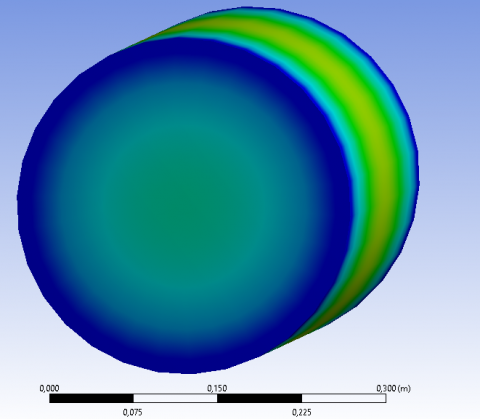Figure 10. Temperature profile through a cylindrical cross section of a NiTi SMA without heat sink

## image036.png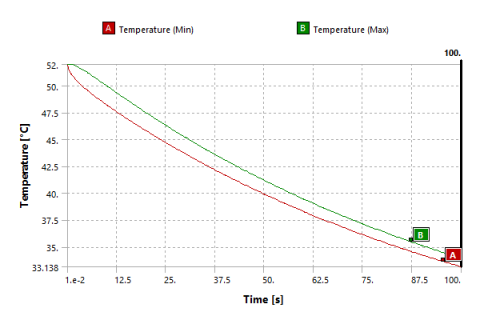Figure 11. Temperature-time graph for a Nitinol SMA without heat sink

3.2 Total heat flux of SMA without heat sink

The rate at which thermal energy is transmitted across a unit surface per unit of time is defined as heat flux. It is one of the parameters that must be considered in heat transfer calculations. Because it has both direction and magnitude, it is a vector quantity. As shown in Figure 12, it was found to be growing from the center to the outer corners of the SMA. The minimal value is found in the center, while the highest value is found on the outer edges.

## image037.png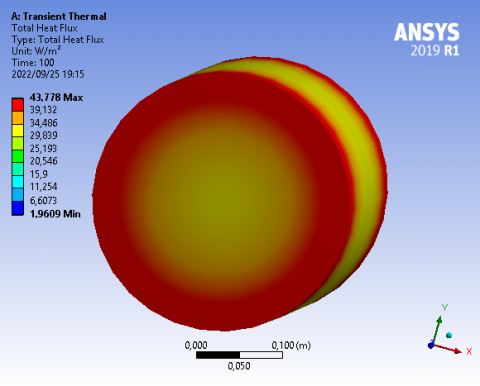Figure 12. Profile of total heat flux for a cylindrical cross section of a Nitinol SMA without heat sink

This implies that heat is carried from the center of the SMA to the outer edges, such that after 37 seconds, the reverse phase change occurs, and the center is cooled as the heat is dispersed, with much of it accumulating on the SMA's outer edges. The pace at which heat is dissipated from the center of the SMA to the outer edges is assumed to be directly related to the response time of the robotic gripper's fingers.

Heat flow as seen in Figure 12 appears to be compatible with the total heat flux profile in a Nitinol SMA shown in Figure 13, in which heat is transferred from the center of the SMA to the outside borders, where it should be dissipated to the ambient environment. It is discovered that the outer corners of the SMA experience the highest heat, which diminishes as we get closer to the center. We can derive the following formula for heat flux from energy balance.

$\frac{\partial {E}_{\text {in }}}{\partial t}-\frac{\partial {E}_{\text {out }}}{\partial t}-\frac{\partial {E}_{\text {accumulated }}}{\partial t}\quad=0$                               (19)

where, $\frac{\partial E_{i n}}{\partial T}$ is the time rate of change of the total amount of incoming energy, $\frac{\partial E_{\text {out }}}{\partial t}$ is the time rate of change of total amount of outgoing energy and $\frac{\partial E_{\text {accumulated }}}{\partial t}\quad$ is the time rate of change of total amount of accumulated energy.

If heat transfer is the only means by which a system exchanges energy with its surroundings, the energy balance can be calculated by using heat transfer, as illustrated by Eq. (20).

$\frac{\partial {E}_{\text {in }}}{\partial t}-\frac{\partial {E}_{\text {out }}}{\partial t}=\oint_S \vec{Q} \cdot \overrightarrow{d s}$                               (20)

where, the heat flux Q has been integrated over the system's surface area S. The overall heat flux, as shown in Figure 12, increases abruptly and then begins to decline over time. As a result, the total heat flux begins at a high rate and gradually decreases over time. Because the zone of thermal equilibrium has not yet been approached, the rate of heat transfer is initially at its highest. The zone of thermal equilibrium is approached over time, and the rate of heat transmission decreases.

## image043.png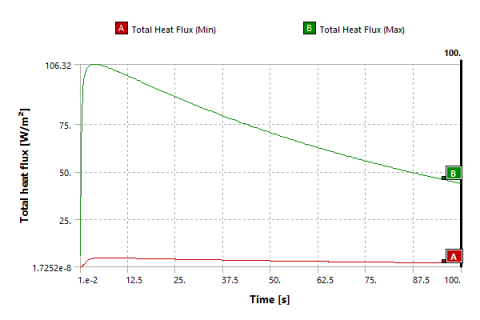Figure 13. Total heat flux vs time for a Nitinol SMA without heat sink

3.4 Temperature distribution of SMA coupled with heat sink

NiTi SMA is connected to a heat sink consisting of a silicone exterior housing and thermal compound. A thermal compound has excellent heat conductivity and can improve conduction by enhancing the thermal coupling between surfaces. It is also known as thermal paste, heat sink paste, heat sink compound, and thermal interface material, among other things. Surfaces in touch have contact only at discrete locations, with minimal surface contact, due to surface imperfections. The thermal compound binds to surfaces and forms a sealant between discrete contact sites, resulting in maximal surface contact and, as a result, a better heat conduction path than contact points alone. The heat sink is predicted to facilitate heat dissipation from the heated SMA actuator; thus, the reverse martensitic phase transformation should take less time than the SMA without a heat sink. Figure 14 illustrates heat flow from the embedded SMA to the outer compliant layer. In correlation with Figure 15, it is observed that after 24 s most of the heat is concentrated in the outer layer while the SMA remains relatively cool.

## image044.pngFigure 14. Temperature distribution through a cylindrical cross section of a heat sink coupled SMA

## image045.png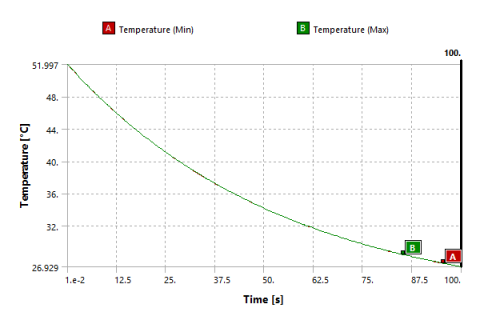Figure 15. Temperature vs time for a heat sink coupled SMA

For a heat sink coupled SMA, a time observed for reverse phase transformation is about 24 s. That is a 13 s decrease from the 37 s observed when the SMA was not coupled with a heat sink and subject to ambient conditions. The average response time is then 30.5 s. The percentage difference between the response time observed without heat sink and that observed with heat sink is then 42%, this constitutes a 42% improvement in the response time when a heat sink is used.

3.5 Total heat flux

The slope of the graph of rate of total heat flux for an SMA coupled with a heat sink as illustrated by Figure 14, is much steeper than that of an SMA without a heat sink depicted by Figure 12. This implies a faster rate of heat transfer from the embedded SMA to the ambient environment in the presence of heat sink as compared to without. This is supported by observing that when a heat sink is incorporated, we have a total heat flux of 50 W/m2 after a time of 37.5 s as illustrated by Figure 16. Whereas without a heat sink, we observe the same total heat flux of 50 W/m2 after 87.5 s as illustrated by Figure 12. What this means is that in a short space of time more heat has been dissipated from the SMA when coupled with a heat sink than when not.

## image046.png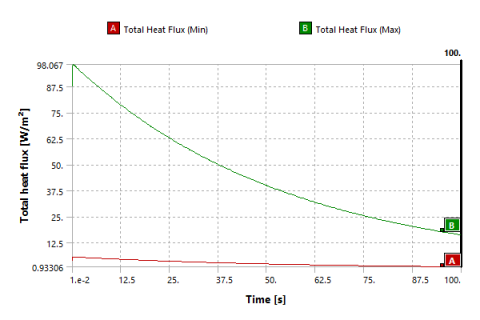Figure 16. Total heat flux vs time for a heat sink coupled SMA

3.7 Experimental results

When the heat was dissipated without the use of a heat sink, the experimental findings showed an average cooling time of 98 seconds. Table 7 displays the cooling time findings from five experimental trials.

According to the experimental data, an average cooling time of 98 seconds was observed during the cooling process in the absence of a heat sink. The time was slightly longer than that reported in the numerical findings, this could be explained by the mesh generated during the simulation procedure. A finer mesh would have yielded results much more closely related to those obtained experimentally.

The experimental results showed that the cooling process with a heat sink took an average of 87 seconds. That is an 11-second difference in cooling time noticed when the heat sink was present versus when it was not. It amounts to an average of 92.5 seconds and a 10.2 percent percentage difference.

The numerical data showed a 13 s reduction in response time, representing a 36% improvement in response time, whereas the experimental results showed an 11-second decrease in response time, representing a 10.2% improvement in response time. The disparity could be explained by the mesh generated during the numerical simulations. A finer mesh would have brought the numerical results considerably closer to the experimental results.

Table 7. Experimental results for the cooling process with and without a heat sink

 Results for the Cooling Process Without Heat Sink Trial Cooling Time Observed (s) Trial 1 99 Trial 2 99 Trial 3 97 Trial 4 97 Trial 5 98 Average cooling time 98 Results for the Cooling Process with Heat Sink Trial Cooling Time Observed (s) Trial 1 88 Trial 2 86 Trial 3 86 Trial 4 87 Trial 5 88 Average cooling time 87
4. Conclusion and Future Research Work

This study aimed to analyze the response time of an SMA-based soft robotic gripper with variable stiffness, with the goal to improve upon it. The results showed that the heat sink improves the response time of the SMA-actuated soft robotic gripper by up to 42%. This demonstrates the potential of a simple heat sink-based approach to enhance the actuation speed of SMA-driven soft robotics. The results indicate that a simple and cost-effective heat sink can be fabricated, which maintains the lightweight and compact structure of the soft robotic gripper. The findings of this study contribute towards the improvement of the response time of SMA based soft robotic grippers with variable stiffness. This study investigated a simple solution to the problem of a slow response time of SMA-based soft robotic grippers with variable stiffness. The limitation of this study is that the SMA used was of large dimension, and therefore was not able to accurately mimic the SME characteristic of SMAs. To overcome this limitation, an SMA with smaller dimensions can be used in further studies. Future work includes studying the ways to alter the properties of SMA actuators with the goal of achieving a more rapid response time; exploring other materials that maintain the simplicity and cost-effectiveness of the design while attempting to further improve upon the response time; and investigating porous heat sinks, flexible heat pipes or other conductive composites that may provide even faster heat dissipation.

References

 She, Y., Li, C., Cleary, J., Su, H.J. (2015). Design and fabrication of a soft robotic hand with embedded actuators and sensors. Journal of Mechanisms and Robotics, 7(2): 021007. https://doi.org/10.1115/1.4029497

 Wang, W., Ahn, S.H. (2017). Shape memory alloy-based soft gripper with variable stiffness for compliant and effective grasping. Soft Robotics, 4(4): 379-389. https://doi.org/10.1089/soro.2016.0081

 Kim, S., Laschi, C., Trimmer, B. (2013). Soft robotics: A bioinspired evolution in robotics. Trends in Biotechnology, 31(5): 287-294. https://doi.org/10.1016/j.tibtech.2013.03.002

 Schäffer, A.A., Eiberger, O., Grebenstein, M., Haddadin, S., Ott, C., Wimböck, T., Hirzinger, G. (2008). Soft robotics, from torque feedback-controlled lightweight robots to intrinsically compliant systems. IEEE Robotics and Automation Magazine, 15(3): 20-30.

 Cheng, N.G., Gopinath, A., Wang, L., Iagnemma, K., Hosoi, A.E. (2014). Thermally tunable, self-healing composites for soft robotic applications. Macromolecular Materials and Engineering, 299(11): 1279-1284. https://doi.org/10.1002/mame.201400017

 Jiang, A., Aste, T., Dasgupta, P., Althoefer, K., Nanayakkara, T. (2013). Granular jamming with hydraulic control. In International Design Engineering Technical Conferences and Computers and Information in Engineering Conference, 55935: V06AT07A021. https://doi.org/10.1115/DETC2013-12213

 Velázquez, R., Pissaloux, E.E. (2012). Modelling and temperature control of shape memory alloys with fast electrical heating. International Journal of Mechanics and Control, 13(2): 1-8.

 Mehta, K., Gupta, K. (2019). Fabrication and Processing of Shape Memory Alloys (pp. 6-7). Cham: Springer International Publishing. https://doi.org/10.1007/978-3-319-99307-2

 Sampath, S., Vedamanickam, S. (2023). Effect of vanadium on the microstructure, transformation temperatures, and corrosion behavior of NiTi shape memory alloys. Journal of Engineering Materials and Technology, 145(1): 011008. https://doi.org/10.1115/1.4055910

 Stöckel, D. (1995). The shape memory effect-phenomenon, alloys and applications. Proceedings: Shape Memory Alloys for Power Systems EPRI, 1: 1-13.

 Santosh, S., Thomas, J.K., Pavithran, M., Nithyanandh, G., Ashwath, J. (2022). An experimental analysis on the influence of CO2 laser machining parameters on a copper-based shape memory alloy. Optics & Laser Technology, 153: 108210. https://doi.org/10.1016/j.optlastec.2022.108210

 Santosh, S., Praveen, R., Sampath, V. (2019). Influence of cobalt on the hot deformation characteristics of an NiTi shape memory alloy. Transactions of the Indian Institute of Metals, 72: 1465-1468. https://doi.org/10.1007/s12666-019-01591-6

 Otsuka, K., Wayman, C. (1998). Shape Memory Materials. Cambridge: The Press Syndicate of the University of Cambridge.

 Lewis, F., Dawson, D., Abdallah, C. (2004). Control Engineering A series of Reference Books and Texbooks. In N. Munro, & F. Lewis (A cura di), Robot manipulator control theory and practice (p. 1-607). NY: Marcel Dekker.Inc.

 Balasubramaniyan, C., Rajkumar, K., Santosh, S. (2022). Enhancement of machining and surface quality of quaternary alloyed NiTiCuZr shape memory alloy through ultrasonic vibration coupled WEDM. Proceedings of the Institution of Mechanical Engineers, Part L: Journal of Materials: Design and Applications, 236(4): 816-833. https://doi.org/10.1177/14644207211058297

 Connor, N. (2019). What is thermal resistance – Thermal resistivity – Definition. https://www.thermal-engineering.org/what-is-thermal-resistance-thermal-resistivity-definition/, accessed on January 20, 2023.

 Khan, L.A., McCarthy, E., Muilwijk, C., Ahad, I.U., Brabazon, D. (2023). Analysis of nitinol actuator response under controlled conductive heating regimes. Results in Engineering, 18: 101047. https://doi.org/10.1016/j.rineng.2023.101047

 Kosky, P., Balmer, R., Keat, W.D., Wise, G. (2010). Exploring Engineering. Second Edition: An Introduction to Engineering and Design. Academic Publishing, Oxford, UK.

 Coyle, S., Majidi, C., LeDuc, P., Hsia, K.J. (2018). Bio-inspired soft robotics: Material selection, actuation, and design. Extreme Mechanics Letters, 22: 51-59. https://doi.org/10.1016/j.eml.2018.05.003

 Schaller, C. (2022). Polymer Chemistry. LibreTexts.

 Han, R., Li, Y., Zhu, Q., Niu, K. (2022). Research on the preparation and thermal stability of silicone rubber composites: A review. Composites Part C: Open Access, 8: 100249. https://doi.org/10.1016/j.jcomc.2022.100249

 Shin-Etsu. (2020). Charecteristic properties of silicone rubber compounds. https://www.shinetsusilicone-global.com/catalog/pdf/rubber_e.pdf, accessed on January 20, 2023.

 Wordenweber, B. (1984). Finite Element Mesh Generation. Cambridge, UK: Butterworth & Co (Publishers) Lid.

 Industrial Rubber Goods. (2022). Silicone Rubber (Si Rubber). http://www.industrialrubbergoods.com/silicone-rubber.html, accessed on January 20, 2023.